# 5 Most Common Motor Load Types

2016-03-25
Load profile (speed range, torque and power)

Certain load types are characteristic in the industrial world. Knowing the load profile (speed range, torque and power) is essential when selecting a suitable motor and frequency converter for the application.

Some common load types are shown. There may also be combinations of these types.

1. Constant Torque
3. Constant power
4. Constant power/torque
5. Starting/breakaway torque demand

1. Constant torque

A constant torque load type is typical when fixed volumes are being handled. For example screw compressors, feeders and conveyors are typical constant torque applications.

Torque is constant and the power is linearly proportional to the speed.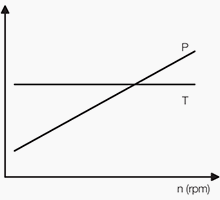Typical torque and power curves in a constant torque application
Figure 1 – Typical torque and power curves in a constant torque application

Quadratic torque is the most common load type. Typical applications are centrifugal pumps and fans. The torque is quadratically, and the power is cubically proportional to the speed.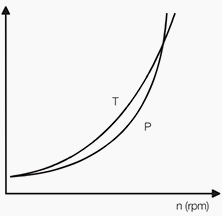Typical torque and power curves in a quadratic torque application
Figure 2 – Typical torque and power curves in a quadratic torque application

3. Constant power

A constant power load is normal when material is being rolled and the diameter changes during rolling. The power is constant and the torque is inversely proportional to the speed.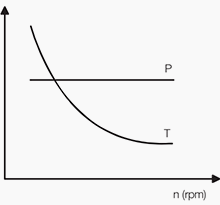Typical torque and power curves in a constant power application
Figure 3 – Typical torque and power curves in a constant power application

4. Constant power/torque

This load type is common in the paper industry. It is a combination of constant power and constant torque load types. This load type is often a consequence of dimensioning the system according to the need for certain power at high speed.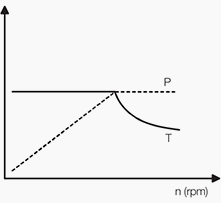Typical torque and power curves in a constant power/torque application
Figure 4 – Typical torque and power curves in a constant power/torque application

5. Starting/breakaway torque demand

In some applications high torque at low frequencies is needed. This has to be considered in dimensioning. Typical applications for this load type are for example extruders and screw pumps.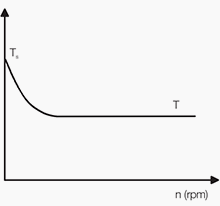Typical torque curve in an application where starting torque is needed
Figure 5 – Typical torque curve in an application where starting torque is needed

Product Categories